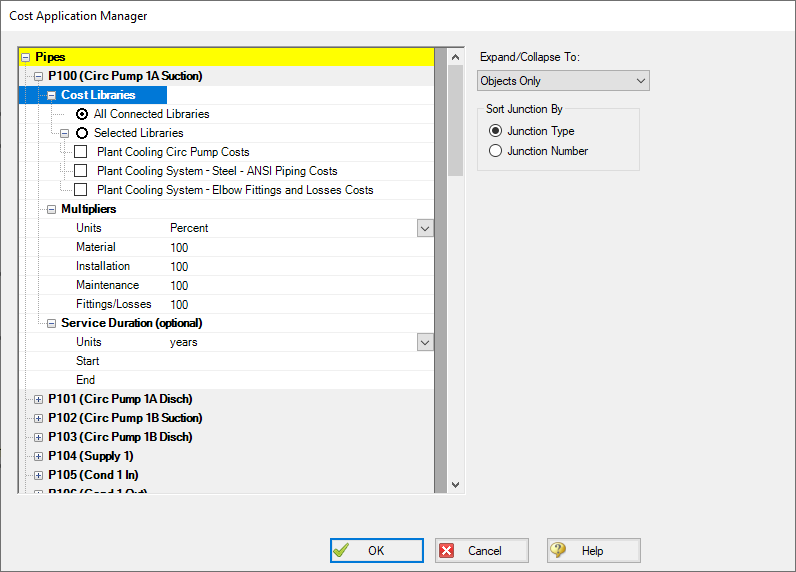## Cost Application Manager

Note:: This topic does not apply for the ANS module. To set up cost calculations with ANS, see the Assign Cost Libraries topic.

The Cost Application Manager, accessed from an object's Cost tab, allows the user to do the following:

• Choose which cost libraries will be applied to each pipe and junction

• Note that if multiple libraries are applied, costs from ALL of the selected libraries will be included.

• Choose when each pipes or junctions life will start and/or end

• Specify fractional cost multipliers to be applied (for cases such as pump energy when the pump operates only part of the time)

The Cost Application Manager can also be accessed from the Tools menu - in which case it will show data for all Workspace objects.Figure 1: Cost Application Manager

How Duplicate Costs are Handled

To maximize flexibility for the user, multiple cost libraries can be connected to the same engineering library. What happens if there is cost data for the same engineering item in each library? For example, assume an engineering library, pump.dat, exists with a specific pump "PumpCo Model 100A".

Two cost libraries exist based on pump.dat:

• maxcost.cst ("PumpCo Model 100A" installation cost = \$1000)

• bestcost.cst ("PumpCo Model 100A" installation cost = \$750)

If both of these libraries are connected, and All Connected Libraries are applied to the pump, AFT Fathom will interpret the cost for "PumpCo Model 100A" as the sum of the two: \$1750.

There will be occasions you want cost items to be summed, and other cases where you do not. Obviously, if you have two libraries, one that tracks the maximum cost and the other that tracks the "best" cost of an item, you want to use only one cost at a time and not have the costs summed. This can be accomplished with the Cost Application Manager, by selecting only the desired library to apply.

However, perhaps the two acquisition costs do not represent the same item, but rather represent partial costs of that item that you want to maintain separately. For example, one library may contain costs of the pump and its casing, while another may contain cost for the motor. A third might contain cost for a variable speed drive. When managed in this way, you want the costs to be summed.